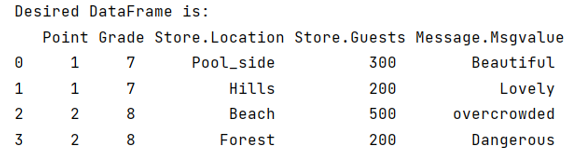# Convert Dataframe column of list with dictionaries into separate columns and expand Dataframe

Given a Pandas DataFrame, we have to convert its column of list with dictionaries into separate columns and expand it. By Pranit Sharma Last updated : September 24, 2023

Pandas is a special tool that allows us to perform complex manipulations of data effectively and efficiently. Inside pandas, we mostly deal with a dataset in the form of DataFrame. DataFrames are 2-dimensional data structures in pandas. DataFrames consist of rows, columns, and data.

Columns are the different fields that contain their particular values when we create a DataFrame. We can perform certain operations on both rows & column values.

## Converting Dataframe column of list with dictionaries into separate columns and expand Dataframe

For this purpose, we will first create a nested dictionary, then we will create the DataFrame by normalizing the JSON format (the nested dictionary) with its specific keys. We will then define the columns which we want as separate columns and expand DataFrame.

Note

To work with pandas, we need to import pandas package first, below is the syntax:

```import pandas as pd
```

Let us understand with the help of an example,

## Python program to convert Dataframe column of list with dictionaries into separate columns and expand Dataframe

```# Importing pandas package
import pandas as pd

# Creating a nested dictionary which can be
# in the form of JSON
d = [
{
'Point':1,
'Store':[{'Count':{'Location': 'Pool_side', 'Guests': '300'}, 'Message':{'Msgvalue':'Beautiful'}},{'Count':{'Location': 'Hills', 'Guests': '200'}, 'Message':{'Msgvalue':'Lovely'}}]
},
{
'Point':2,
'Store':[{'Count':{'Location': 'Beach', 'Guests': '500'}, 'Message':{'Msgvalue':'overcrowded'}},{'Count':{'Location': 'Forest', 'Guests': '200'}, 'Message':{'Msgvalue':'Dangerous'}}]
}
]

# Normalising JSON and then creating DataFrame

df = df[df.columns.tolist()[-2:] + df.columns.tolist()[:-2]]

# Assigning columns
df.columns = [c.replace('Count','Store') for c in df.columns]

# Display DataFrame
print("Desired DataFrame is:\n",df)
```

### Output

The output of the above program is: